# 彻底搞懂++i,i++再也不迷糊``````public class test1 {
public static void main(String[] args) {
int i = 1;
i = i++;
int j = i++;
int k = i + ++i * i++;
System.out.println("i="+i);
System.out.println("j="+j);
System.out.println("k="+k);
}
}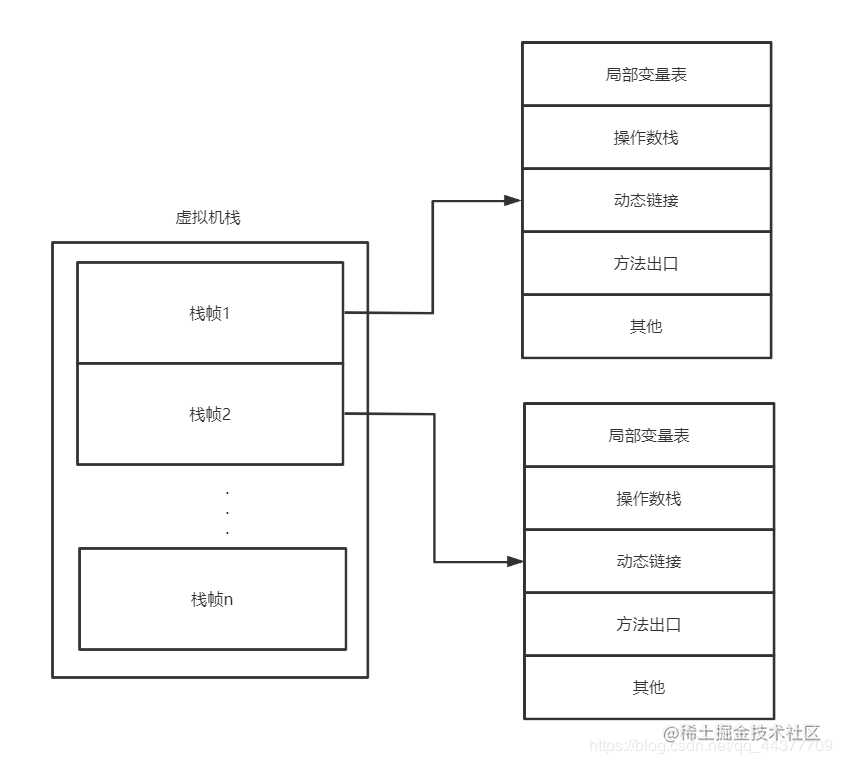此题目我们只需要用到栈帧里面的局部变量表和操作数栈

• 2.1、第一步
``````int i = 1;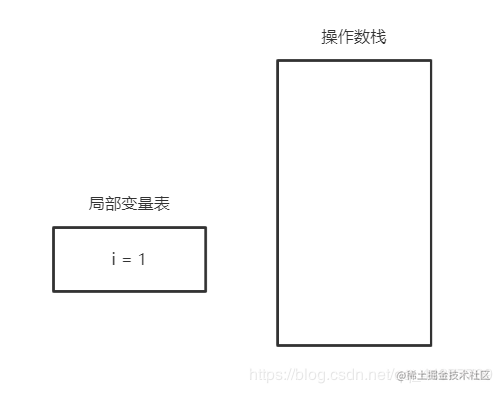只是一个简单的赋值操作

• 2.2、第二步
``````i = i++;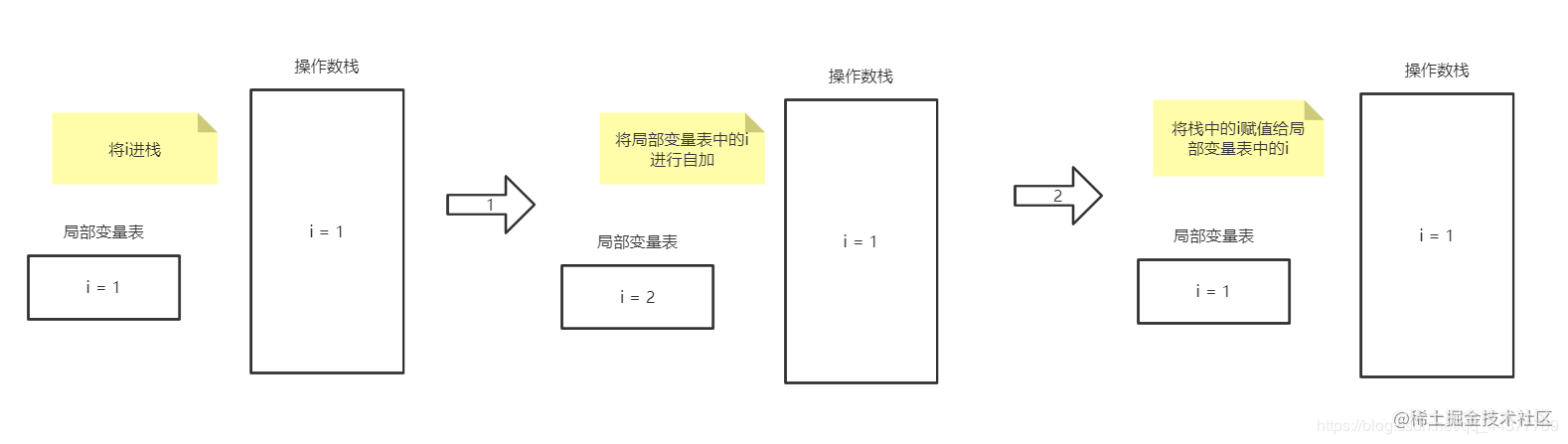结果：i还是等于1

• 2.3、第三步
``````int j = i++;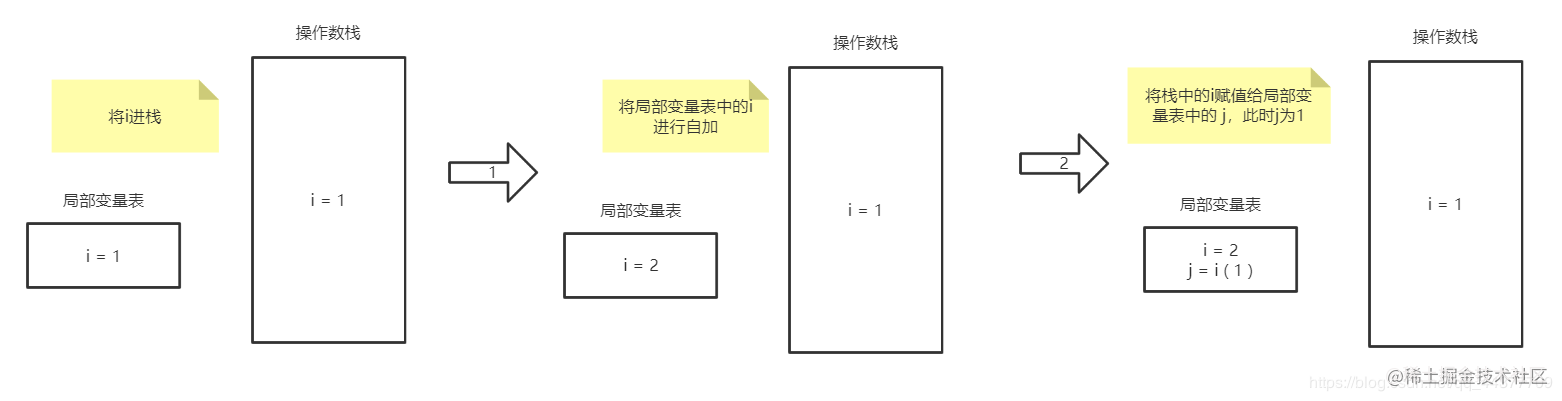结果：i在局部变量表中变成了2，操作数栈中的 i 值为1，并且将i的值返回给j，即此条语句以后，`i = 2,j = 1`

• 2.4、第四步
``````int k = i + ++i * i++;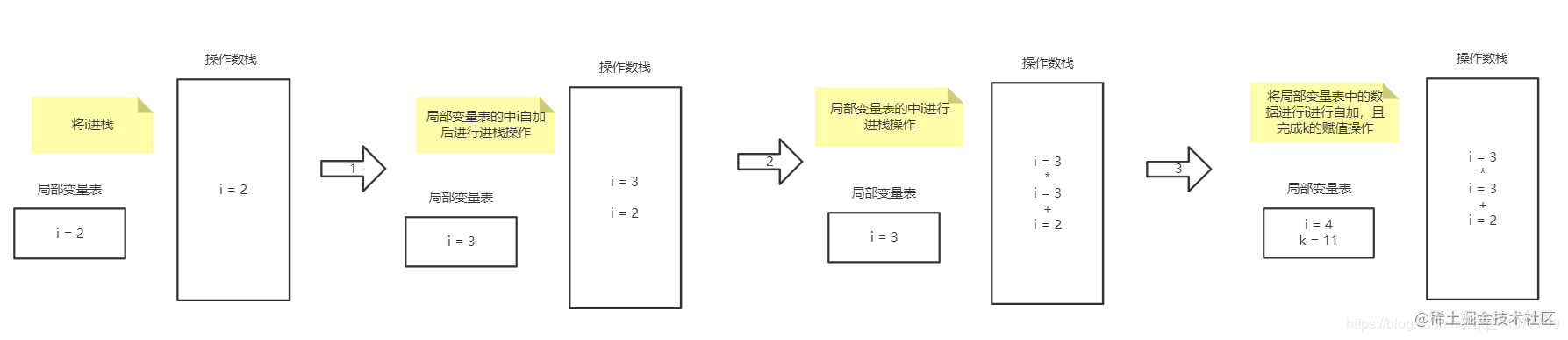结果：局部变量表中的i = 4，k = 11

• 2.5、结果
``````i=4
j=1
k=11

## 三、i = ++i

``````public class test2 {
public static void main(String[] args) {
int i = 1;
i = ++i;
System.out.println(i);
// 结果：i = 2
}
}

blog.csdn.net/qq_44377709…